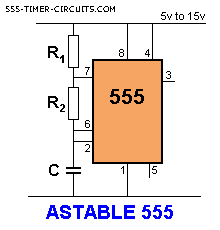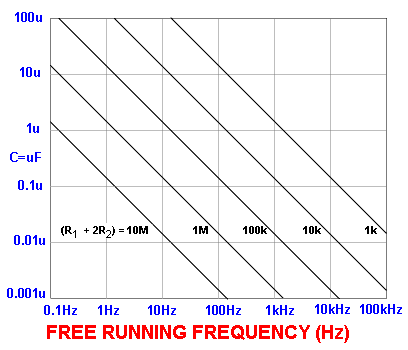# 555 Timer Circuits

555 Timers are fun and a great way to start learning electronics

## THE ASTABLE (or FREE RUNNING) MULTIVIBRATOR CircuitThe capacitor C charges via R1 and R2 and when the voltage on the capacitor reaches 2/3 of the supply, pin 6 detects this and pin 7 connects to 0v. The capacitor discharges through R2 until its voltage is 1/3 of the supply and pin 2 detects this and turns off pin 7 to repeat the cycle.

The top resistor is included to prevent pin 7 being damaged as it shorts to 0v when pin 6 detects 2/3 rail voltage.

Its resistance is small compared to R2 and does not come into the timing of the oscillator.

The  following graph applies to the Astable circuit:Using the graph:

Suppose R1 = 1k, R2 = 10k and C = 0.1u (100n).

Using the formula on the graph, the total resistance  = 1 + 10 + 10 = 21k

The scales on the graph are logarithmic so that 21k is approximately near the "1" on the 10k. Draw a line parallel to the lines on the graph and where it crosses the 0.1u line, is the answer. The result is approx 900Hz.

Suppose R1 = 10k, R2 = 100k and C = 1u

Using the formula on the graph, the total resistance  = 10 + 100 + 100 = 210k

The scales on the graph are logarithmic so that 210k is approximately near the first "0" on the 100k. Draw a line parallel to the lines on the graph and where it crosses the 1u line, is the answer. The result is approx 9Hz.

The frequency of an astable circuit can also be worked out from the following formula:

frequency =            1.4
(R1 + 2R2) Î C
 555 astable frequencies C R1 = 1k R2 = 6k8 R1 = 10k R2 = 68k R1 = 100k R2 = 680k 0.001Á 100kHz 10kHz 1kHz 0.01Á 10kHz 1kHz 100Hz 0.1Á 1kHz 100Hz 10Hz 1Á 100Hz 10Hz 1Hz 10Á 10Hz 1Hz 0.1Hz

Comment on this circuit or view the Electronic Circuits Community Forum.